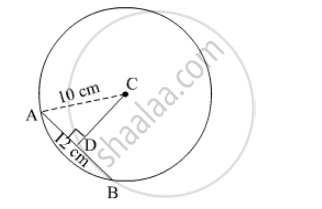# C is the Centre of the Circle Whose Radius is 10 Cm. Find the Distance of the Chord from the Centre If the Length of the Chord is 12 Cm. - Mathematics

Sum

C is the centre of the circle whose radius is 10 cm. Find the distance of the chord from the centre if the length of the chord is 12 cm.

#### SolutionC is the centre of the circle.
Let the chord be AB.
Distance of the centre to the chord = AB.
CD is perpendiculaar to the chord AB.
Perpendicular drawn from the centre of the circle to the chord bisects the chord.

AD = "AB"/2 = 12/2 = 6 cm

In Δ ACD,
We apply the Pythagoras theorem

⇒ CD² + 6² = 10²

⇒ CD² + 36² = 100

⇒ CD² = 64

⇒ CD = 8 cm

Thus, distance of the chord from the centre is 8 cm.

Concept: Properties of Chord of a Circle
Is there an error in this question or solution?

#### APPEARS IN

Balbharati Mathematics 8th Standard Maharashtra State Board
Chapter 17 Circle : Chord and Arc
Practice Set 17.1 | Q 4 | Page 116